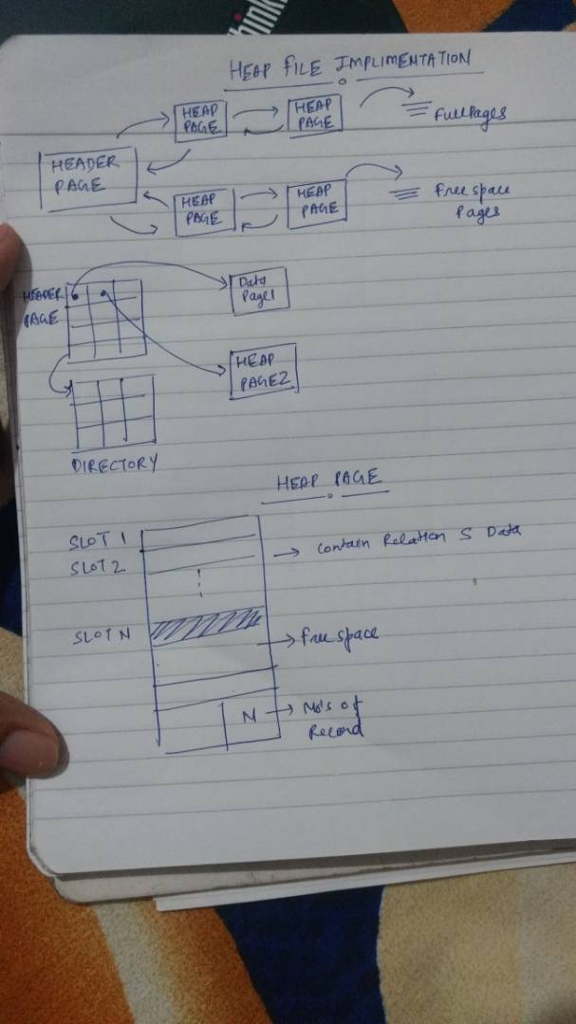# Answered! (Storage) Consider a relation S with the following schema: S(a int, b float, c char(1)). Consider the following small instance of S:…

(Storage) Consider a relation S with the following schema: S(a int, b float, c char(1)). Consider the following small instance of S:

Table S

Don't use plagiarized sources. Get Your Custom Essay on
Answered! (Storage) Consider a relation S with the following schema: S(a int, b float, c char(1)). Consider the following small instance of S:…
GET AN ESSAY WRITTEN FOR YOU FROM AS LOW AS \$13/PAGE

A B C

1 57.0 F

2 12.0 S

3 4.0 T

Assume that S is stored in a heap file on disk. Assume also a page size of 8KB (8192 bytes), 4-byte integers, and 8-byte floats.

Draw a schematic representation of the Heap file page on disk storing the S instance.

The size of each S tuple in bytes is 4+8+20 or 32 bytes. How many S tuples fit on one page? Assume a page header is 20 bytes. How many pages are necessary to hold an instance of S with 1000 tuples (remember the space necessary for the page header)?

Perform the following sequence of operations drawing schematic representations at each step.

Insert(4,14.8, F);

Delete(2);

Update(1) to (1,59.0, F)